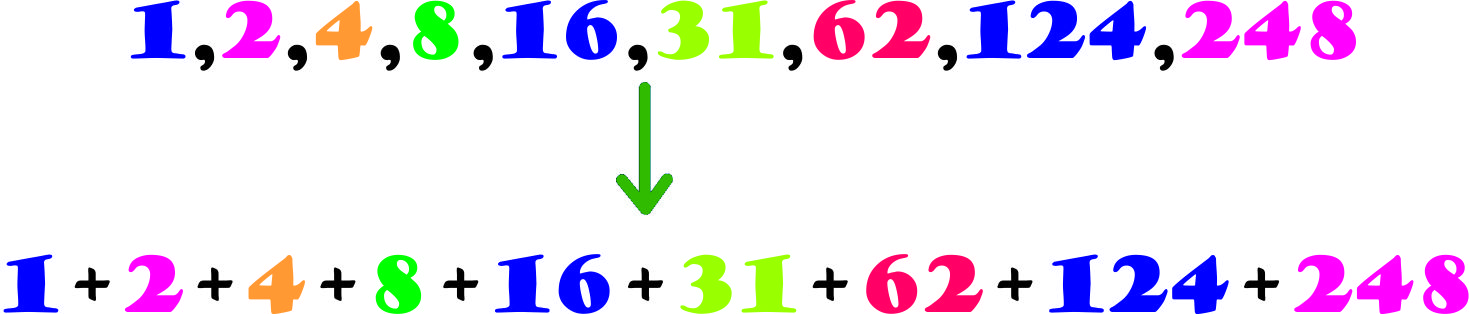# Are you Perfect or Superperfect?How many Perfect Numbers are there which are also Super Perfect?

Details and Assumptions

$n$ is called a superperfect number when $\sigma(\sigma(n))=2n$

$\sigma(n)$ denotes the sum of the divisors of $n$ (from $1$ to $n$ inclusive).

###### Image Credit: Wikimedia Perfect Number by Deryni
×

Problem Loading...

Note Loading...

Set Loading...